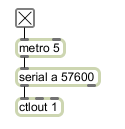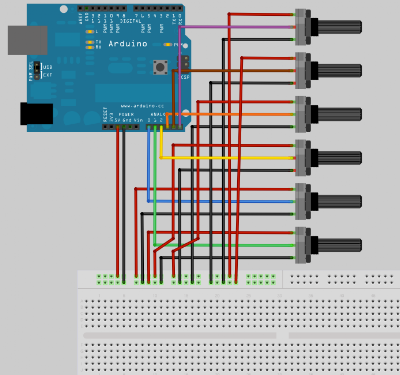## Saturday, August 27, 2011

### Simple Examples of Sending MIDI Data from Arduino to Computer

Sending a Single Stream of Data
It's easy to send data from just one sensor or button on the Arduino to Max/MSP for further processing and routing to music applications. Take the following example, which reads a potentiometer from Arduino analog input pin 0 and sends this data to Max/MSP as a serial stream of bytes. This stream of bytes has a data range of 0 - 127, perfect for MIDI control applications.

Once the data has been received in Max/MSP, it can be routed to a ctlout object, thus allowing control of any parameter in any application that accepts MIDI continuous controller inputs.

Note that you may want to copy and paste the Arduino code examples into the Arduino programming environment and then go to Tools > Auto Format (Command or Control T) for legibility.

Arduino Board Hardware:Arduino Board Code:

byte val; // create variable to store data

void setup() {
Serial.begin(57600); // open serial port
}

void loop() {
val = analogRead(0) / 8; // read value from pot and make value from 0 - 127
Serial.print(val, BYTE); // print value to serial port
delay(5); // wait for a brief moment
}

Max/MSP Patch:But what about two, three or four potentiometers? If we want to send data from more than one potentiometer we start to run into trouble. This is because our serial port on the Arduino and on the computer can only send / receive data one byte at a time. So if we want to, for example, send the data from two potentiometers from Arduino to Max/MSP, then we are stuck if we are using a basic serial port set up.

In such a scenario, every first data value that we receive in Max/MSP will belong to one potentiometer and every second data value will belong to the other potentiometer. This then becomes bothersome in terms of pulling these values apart, and reconstructing separate streams.

Solution to Sending Multiple Streams of Data
There are a few ways of getting around this problem. One of the solutions is to format all of our data to conform with MIDI in the Arduino code, so that we can simply send a stream of MIDI formatted data from the Arduino to Max/MSP. This simplifies things on the Max/MSP end, and also allows us to send data from more than one sensor, button etc.

MIDI Formatting
MIDI data formatting may seem strange at first, but it is easy to get the hang of. Every MIDI event (like note-ons, note-offs, pitch bends, controller changes etc) is sent via a MIDI message. For the purpose of the use outlined in this post, we are only going to deal with MIDI messages that contain three bytes. Every byte is made up of eight bits, and is thus a range of values from 0 - 255.

The first byte is the status byte, and the next two bytes are the data bytes. The status byte indicates the kind of data we are sending (like note-ons, note-offs, pitch bends, controller changes etc) and the channel number (from 1 to 16). The data bytes bytes contain the actual information that we are sending, for example if we are sending a note-on event, then our data bytes will contain the pitch of the note and the velocity of the note, like so:

Status Byte; Data Byte #1; Data Byte #2;

Status Byte
The status byte is divided up into two halves called nibbles. The first nibble occupies bits 4 - 7 of the byte, whilst the second nibble occupies bits 0 - 3 of the byte. We can use hexadecimal notation to easily represent this, where the first character is a value from 0 - F (i.e. the first nibble) and the second character is a value from 0 - F (i.e. the second nibble).

The first nibble indicates the type of data we are sending, based on the following table in hexadecimal:

8 = Note Off
9 = Note On
A = After Touch
B = Control Change
C = Patch Change
D = Channel Pressure
E = Pitch Bend
F = System Message

The second nibble of the status byte indicates the channel number, from 1 to 16 as follows:

0 = Channel 1
1 = Channel 2
2 = Channel 3
3 = Channel 4
4 = Channel 5
5 = Channel 6
6 = Channel 7
7 = Channel 8
8 = Channel 9
9 = Channel 10
A = Channel 11
B = Channel 12
C = Channel 13
D = Channel 14
E = Channel 15
F = Channel 16

So if we combine this information, we can form meaningful status bytes. For example, if we want to send a note-on for channel 1, we simply send the value 0x90. The 0x prefix indicates hexadecimal numbering; the 9 indicates that it is a note-on; the 0 indicates that the note on is sent to channel 1. Or, if we want to send a controller change for channel 5, we simply send the value 0xB4. Or, if we want to send a pitch bend for channel 3, we simply send the value 0xE2.

Data Bytes
Once we have sent our status byte, we need to send our two data bytes. These bytes contain the actual information that we are sending. To simplify this post, let's examine two types of data: note-ons and control changes.

If our status byte indicates that we are sending a note-on, then our data byte #1 will contain a pitch value from 0 - 127. Data byte #2 will contain a velocity value from 0 - 127. So, if we want to send a note-on for channel 3 with a pitch of 60 and a velocity of 123, then we need to send the following three bytes:

0x92
60
123

For our status byte (the first value that we sent), the 9 indicates that we are sending a note-on, the 2 indicates that we are sending to channel 3. The next value is our data byte #1, which is 60 in decimal and indicates the pitch of our note on. The third value is our data byte #2, which is 123 in decimal and indicates the velocity of our note on. FYI: A note-on with a velocity of zero is effectively a note-off.

If our status byte indicates that we are sending a controller change, then our data byte 1 will contain a controller number from 0 - 127. Data byte 2 will contain the value of the controller number from 0 - 127. So, if we want to send a controller change message for controller number 1 with a value of 82 on channel 5, then we need to send the following three bytes:

0xB4
1
82

For our status byte (the first value that we sent), the B indicates that we are sending a control change message, the 4 indicates that we are sending to channel 5. The next value is our data byte #1, which is 1 in decimal and indicates the controller number. The third value is our data byte #2, which is 82 in decimal and indicates the actual value of our controller.

Common Controllers
Certain controller numbers are assigned to do certain things, for example:
Modulation (CC #1)
Breath (CC #2)
Foot Pedal (CC #4)
Portamento Time (CC #5)
Volume (CC #7)
Pan (CC #10)
Expression (CC #11)
Soft Pedal (CC #68)

This may come in handy when programming your Arduino to send sensor data as MIDI controllers.

Sending Bytes From Arduino
We can combine the above information and easily send MIDI formatted data from within Arduino code. We can then pick up this formatted data within Max/MSP, and send it along a virtual MIDI path (e.g. IAC Driver Bus 1 in OS X or using LooBe1 in Windows) to our favourite audio application or DAW (e.g. Live, Logic, ProTools, Reason) and control our favourite synth / sequencer / sampler / etc parameters (assuming that they support MIDI control. See below for some basic example of sending a note-on and sending a control change message.

Example 1: Sending a note-on event; waiting for one second; sending a note-off event; waiting for one second

Arduino Board Hardware:Arduino Board Code:
void setup() {
Serial.begin(57600); // open serial port
}

void loop() {
// let's send a note-on
Serial.print(0x90, BYTE); // MIDI Note-on; channel 1
Serial.print(60, BYTE); // MIDI note pitch 60
Serial.print(127, BYTE); // MIDI note velocity 127
delay(1000); // wait for 1 second

// let's send a note-off
Serial.print(0x90, BYTE); // MIDI Note-on; channel 1
Serial.print(60, BYTE); // MIDI note pitch 60
Serial.print(0, BYTE); // MIDI note velocity 0 (i.e. note off)
delay(1000); // wait for 1 second
}

Max/ MSP Patch:Example 2: Sending a control change message every second

Arduino Board Hardware:Arduino Board Code:
void setup() {
Serial.begin(57600); // open serial port
}

void loop() {
// let's send a control change message
Serial.print(0xB2, BYTE); // MIDI control change; channel 3
Serial.print(1, BYTE); // MIDI controller #1
Serial.print(127, BYTE); // MIDI controller value of 127
delay(1000); // wait for 1 second
}

Max/ MSP Patch:Putting It All Together
Now, we can easily read values form multiple potentiometers and send them to Max/MSP as MIDI formatted data. Let's take a look at two examples:

Example 1: Reading the value from two potentiometers connected to analog inputs 1 and 2. These values are sent to MIDI channel 1 controller 1 and MIDI channel 2 controller 1.

Arduino Board Hardware:Arduino Board Code:
byte val = 0;

void setup() {
Serial.begin(57600); // open serial port
}

void loop() {

// let's send a control change message
Serial.print(0xB0, BYTE); // MIDI control change; channel 1
Serial.print(1, BYTE); // MIDI controller #1
Serial.print(val, BYTE); // MIDI controller value from potentiometer 1

// let's send a control change message
Serial.print(0xB1, BYTE); // MIDI control change; channel 2
Serial.print(1, BYTE); // MIDI controller #1
Serial.print(val, BYTE); // MIDI controller value from potentiometer 2
delay(5);
}

Max/ MSP Patch:Example 2: Reading the value from 6 potentiometers and sending the data to controller 1 on channels 1, 2, 3, 4, 5 and 6 respectively.

Arduino Board Hardware:Arduino Board Code:
byte val = 0;

void setup() {
Serial.begin(57600); // open serial port
}

void loop() {
for(int i = 0; i < 6; i ++) {
// depending on which for() loop we are at

// let's send a control change message

Serial.print(0xB0 + i, BYTE); // MIDI control change; channel number
// the channel number depends on which for() loop we ar at
Serial.print(1, BYTE); // MIDI controller #1
Serial.print(val, BYTE); // MIDI controller value from the potentiometer
delay(1); // delay for a brief moment
}
}

Max/ MSP Patch:steve mensink said...

Any thoughts on controlling leds this way?
Perhaps: 'Simple Examples of Sending MIDI Data from Computer to Arduino' ?

steve mensink said...

Any thoughts on controlling leds this way?

Perhaps: "Simple Examples of Sending MIDI Data from Computer to Arduino" ?

Thanks for your article, quite effective info.

Robihn Servant said...

I want to control 2 or more potentiometers using the method described above, but it seems to work only with one pot. I'm using a Wiring 1.1 harware that goes to Max and then to Audiomulch via Loopbe1. Any thoughts on what could go wrong?

rabbitlife@msn.com said...

Have you ever tried using multiple multiplexers in combination with this for more pots?

Anonymous said...

This is very useful in what I'm trying to achieve but how would i achieve the same outcome for push bottons running through the digital inputs on the arduino.

Im new to this and have no real idea on coding. However I am trying and asking questions where I can to better my knowledge.